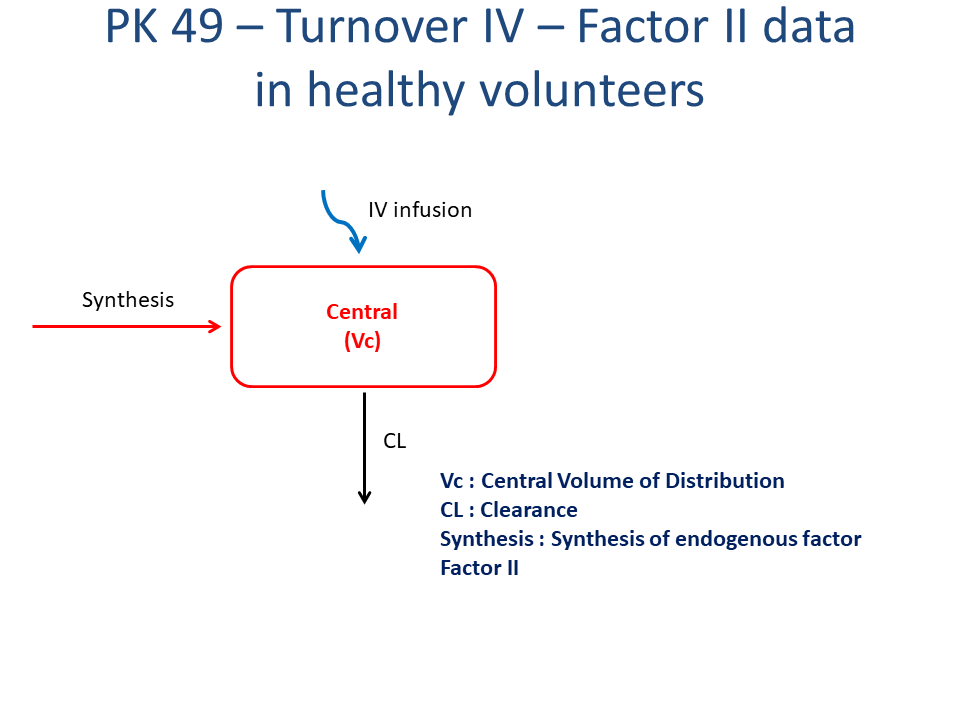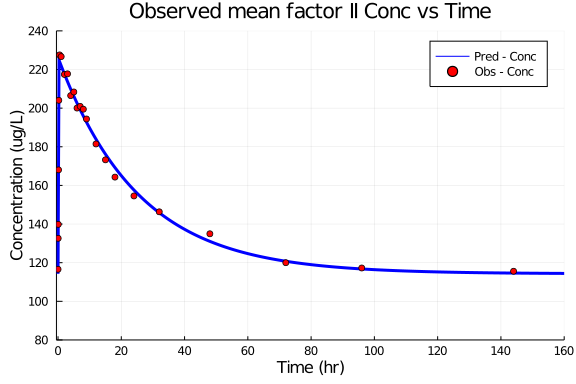# Exercise PK49 - Turnover IV - Factor II data in healthy volunteers

### Background

• Structural model - One compartment turnover model

• Route of administration - Intravenous infusion

• Dosage Regimen - 400 mg dose given as 19 minute constant intravenous infusion

• Number of Subjects - 1 ( Healthy Volunteer )### Objectives

In this tutorial, you will learn how to build One compartment turnover model with constant intravenous infusion and simulate the model for one single subject and single dosage regimen.

### Libraries

call the "necessary" libraries to get started.

using Pumas
using CSV
using StatsPlots
using Plots
using Random


### Model

In this one compartment model, we administer dose on central compartment.

pk_49            = @model begin
@param begin
tvcl         ∈ RealDomain(lower=0)
tvvc         ∈ RealDomain(lower=0)
tvsynthesis  ∈ RealDomain(lower=0)
Ω            ∈ PDiagDomain(3)
end

@random begin
η            ~ MvNormal(Ω)
end

@pre begin
CL           = tvcl * exp(η)
Vc           = tvvc * exp(η)
Synthesis    = tvsynthesis * exp(η)
end

@init begin
Central      = Synthesis/(CL/Vc) #we add Vc here because we want it in "amount". not conc.
end

@dynamics begin
Central'    = Synthesis - (CL/Vc)*Central
end

@derived begin
cp          = @. Central/Vc
end
end

PumasModel
Parameters: tvcl, tvvc, tvsynthesis, Ω, σ_add
Random effects: η
Covariates:
Dynamical variables: Central
Derived: cp, dv
Observed: cp, dv


### Parameters

The parameters are as given below. tv represents the typical value for parameters.

• Cl - Clearance (L/hr)

• Vc - Volume of Central Compartment (L)

• Synthesis - Synthesis of endogenous coagulation Factor II (mg/hr)

• Ω - Between Subject Variability

• σ - Residual error

param = ( tvcl        = 0.14204,
tvvc        = 3.59259,
tvsynthesis = 16.2272,
Ω           = Diagonal([0.0,0.0,0.0]),

(tvcl = 0.14204, tvvc = 3.59259, tvsynthesis = 16.2272, Ω = [0.0 0.0 0.0; 0
.0 0.0 0.0; 0.0 0.0 0.0], σ_add = 2.96)


### Dosage Regimen

A single dose of 400 mg is given as an IV-Infusion over a period of 19 minutes in a healthy individual.

ev1  = DosageRegimen(400, cmt=1, duration=0.3166)
sub1 = Subject(id=1, events=ev1)

Subject
ID: 1
Events: 2


### Simulation

Lets simulate for plasma concentration with the specific observation time points after Intravenous administration.

Random.seed!(123)
sim_sub1 = simobs(pk_49, sub1, param, obstimes=0.00:0.01:160)
df1      = DataFrame(sim_sub1)


### Dataframe and Plot

df1_dv = filter(x -> x.time in [0.01,0.05,0.08,0.16,0.25,0.5,1,2,3,4,5,6,7,8,9,12,15,18,24,32,48,72,96,144], df1)

@df df1 plot(:time, :cp,
title="Observed mean factor II Conc vs Time", label= "Pred - Conc",
xlabel="Time (hr)", ylabel="Concentration (ug/L)", linewidth=3,
xticks=[0,20,40,60,80,100,120,140,160], xlims=(-0.5,160), color=[:blue],
yticks=[0,80,100,120,140,160,180,200,220,240,260,280,300], ylims=(80,240))
@df df1_dv scatter!(:time, :dv, label="Obs - Conc", color=[:red])